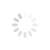## Tips and Resources### Unity - Create a timer clock (minutes & seconds) using Time.timeSinceLevelLoad C#

Converting Time.timeSinceLevelLoad into hours, minutes & seconds using C# and display it as a clock.

Create a TextMeshPro UI object and attach the following script:

``using System.Collections;using System.Collections.Generic;using UnityEngine;using UnityEngine.UI;using TMPro;``public class LevelTimer : MonoBehaviour``{`` TextMeshProUGUI timerText;``// Start is called before the first frame update`` void Start()`` {`` timerText = GetComponentInChildren<TMPro.TextMeshProUGUI>(); // cache the text component`` }``// Update is called once per frame`` void Update()`` {` ` float t = Time.timeSinceLevelLoad; // time since scene loaded``float milliseconds = (Mathf.Floor(t * 100) % 100); // calculate the milliseconds for the timer` ` int seconds = (int)(t % 60); // return the remainder of the seconds divide by 60 as an int`` t /= 60; // divide current time y 60 to get minutes`` int minutes = (int)(t % 60); //return the remainder of the minutes divide by 60 as an int`` t /= 60; // divide by 60 to get hours`` int hours = (int)(t % 24); // return the remainder of the hours divided by 60 as an int``timerText.text = string.Format("{0}:{1}:{2}.{3}", hours.ToString("00"), minutes.ToString("00"), seconds.ToString("00"), milliseconds.ToString("00"));`` }``}``

References: